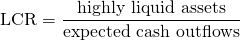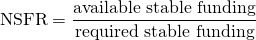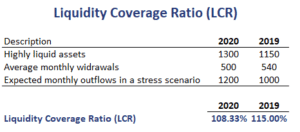# Liquidity Coverage Ratio (LCR)

The liquidity coverage ratio (LCR) is a minimum liquidity standard introduced by Basel III to ensure that banks maintain adequate levels of liquidity. Another liquidity measure that was also introduced by Basel III is the net stable funding ratio (NSFR).

On this page, we discuss both the liquidity coverage ratio definition and the net stable funding ratio definition. We also implement both formulas in Excel using a numerical example. The spreadsheet can be downloaded at the bottom of the page.

## Liquidity coverage ratio formula

Let’s start by discussing the liquidity coverage ratio formulaThus, LCR is defined as the value of the bank’s highly liquid assets divided by its expected cash outflows. Highly liquid assets are assets that can easily be converted to cash. Expected cash outflows are the outflows in a stress scenario. The minimum value should be a 100%.

## Net stable funding ratio formula

The net stable funding ratio (NSFR) equalsThe net stable funding ratio is the percentage of the required stable funding that is obtained from available stable sources of funding. Available stable funding depends on the maturity and composition of the funding sources. The required funding is based on the composition of the assets on the bank’s balance sheet. This value too, should be higher than a 100%.

In this case, the available stable funding (ASF) factor needs to be determined per funding sources. The BIS provides tables that specify the ASF factors for different funding sources.

## Liquidity coverage ratio example

Let’s consider a numerical example. The following table provides data on a bank’s liquid assets and expected cash flows in a stress scenario. Using this information, we calculate the LCR.